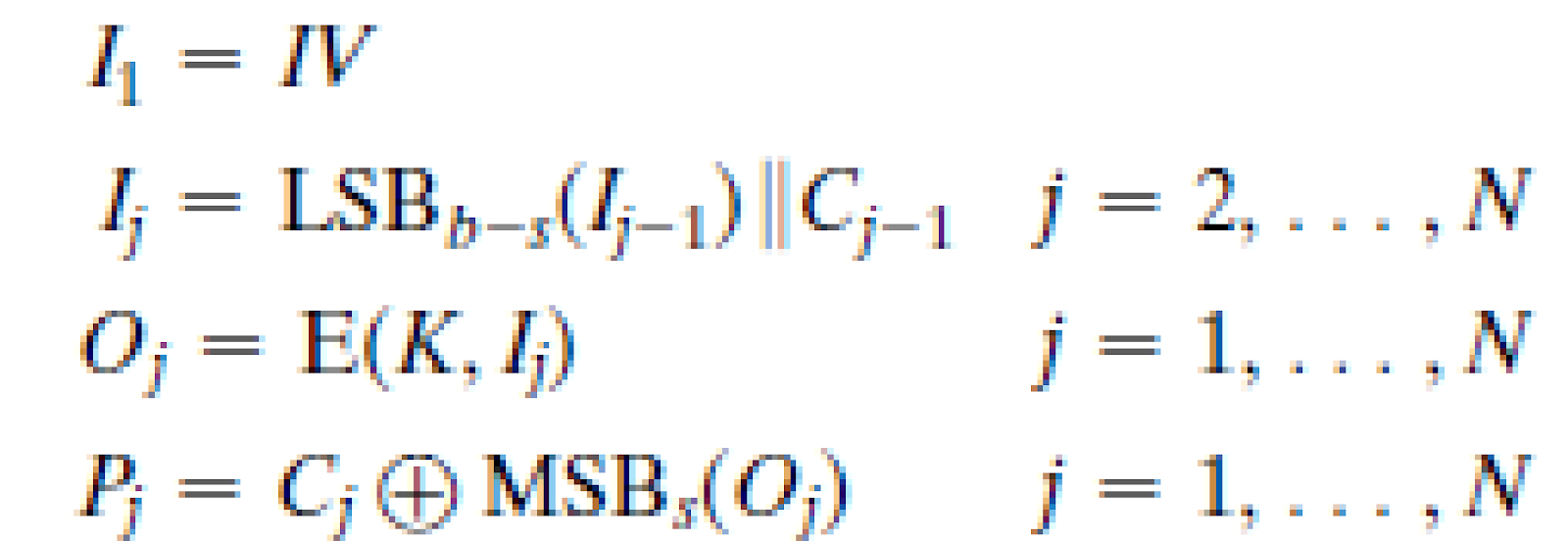# What is Cipher Feed Back mode (CFB)?

Ciphertext feedback (CFB) is a mode of operation for a block cipher. In contrast to the cipher block chaining (CBC) mode, which encrypts a set number of bits of plaintext at a time, it is at times desirable to encrypt and transfer some plaintext values instantly one at a time, for which ciphertext feedback is a method

Cipher Feedback Mode (CFB), Output Feedback (OFB) Mode and Counter(CTR) mode

• These three modes make it possible to convert a block cipher into a stream cipher
•  A stream cipher eliminates the need to pad a message to be an integral number of blocks.
•  It also can operate in real time. ( that is when a character stream is being transmitted, each character can be encrypted and transmitted immediately using a character-oriented stream cipher. In CBC, even if the character is only 8 bits, we have to wait for receiving 64 bits, before starting encryption)

For AES, DES, or any block cipher, encryption is performed on a block of b  bits. In the case of DES, b =  64 and in the case of AES, b =  128. However, it is possible to convert a block cipher into a stream cipher, using one of the three modes to be discussed in this and the next two sections: cipher feedback  (CFB) mode, output feedback  (OFB) mode, and counter  (CTR) mode.

3. Cipher Feedback(CFB) mode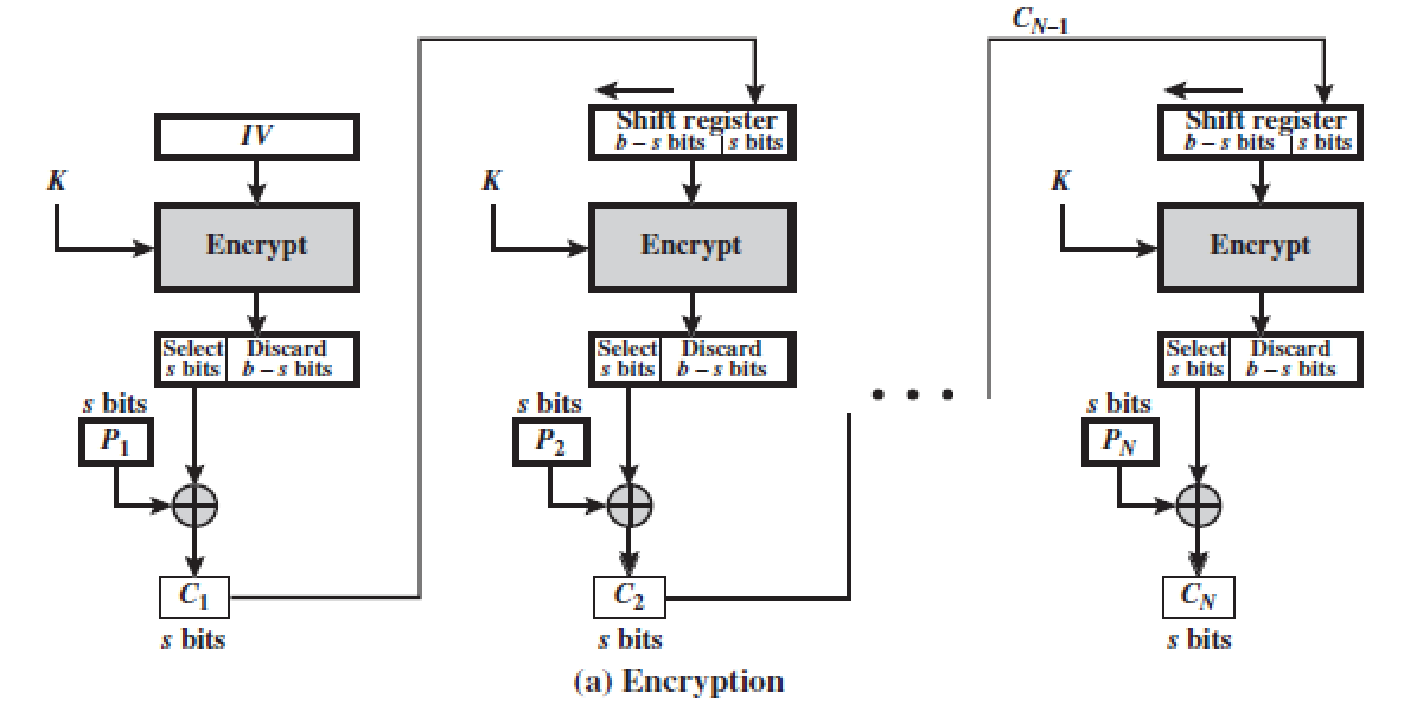Key K and the initialisation vector IV are of b bits length.

Plain text block is of s bits,

Usually s=8 bits

We can define CFB mode encryption as follows: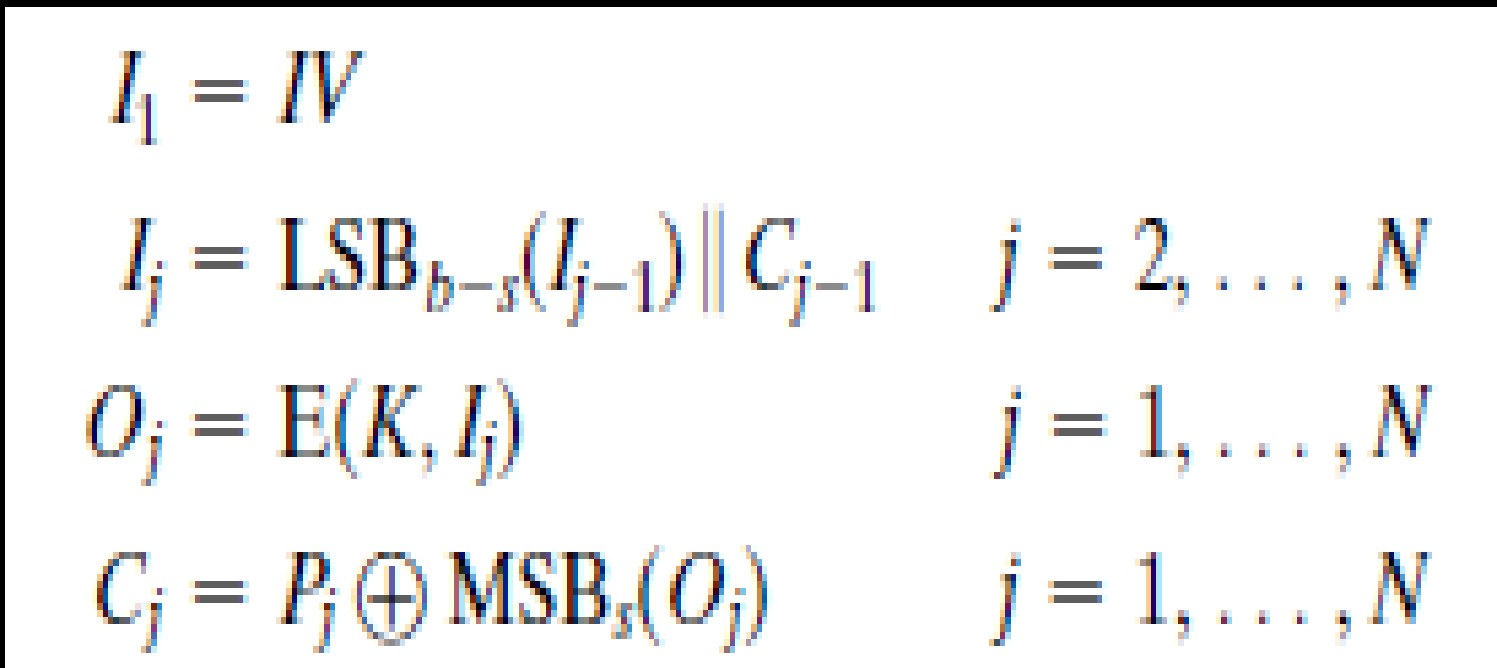Figure 7.5 depicts the CFB scheme. In the figure, it is assumed that the unit of transmission is s  bits; a common value is s =  8. As with CBC, the units of plaintext are chained together, so that the ciphertext of any plaintext unit is a function of all the preceding plaintext. In this case, rather than blocks of b  bits, the plaintext is divided into segments  of s  bits.

First, consider encryption. The input to the encryption function is a b -bit shift register that is initially set to some initialization vector (IV). The leftmost (most significant) s  bits of the output of the encryption function are XORed with the first segment of plaintext P1  to produce the first unit of ciphertext C1 , which is then transmitted. In addition, the contents of the shift register are shifted left by s  bits, and C1  is placed in the rightmost (least significant) s  bits of the shift register. This process continues until all plaintext units have been encrypted.

For decryption, the same scheme is used, except that the received ciphertext unit is XORed with the output of the encryption function to produce the plaintext unit. Note that it is the encryption  function that is used, not the decryption function.  Although CFB can be viewed as a stream cipher, it does not conform to the typical construction of a stream cipher. In a typical stream cipher, the cipher takes  as input some initial value and a key and generates a stream of bits, which is then XORed with the plaintext bits (see Figure 4.1). In the case of CFB, the stream of bits that is XORed with the plaintext also depends on the plaintext.

In CFB encryption, like CBC encryption, the input block to each forward Cipher function (except the first) depends on the result of the previous forward Cipher function; therefore, multiple forward cipher operations cannot be performed in parallel.

In CFB decryption, the required forward cipher operations can be performed in parallel if the input blocks are first constructed (in series) from the IV and the ciphertext.

Main Points:

• The unit of transmission is s bits; a common value is s =  8.
• As with CBC, the units of plaintext are chained together, so that the ciphertext of any plaintext unit is a function of all the preceding plaintext.
• In this case, rather than blocks of b bits, the plaintext is divided into segments of s bits.
• In CFB encryption, like CBC encryption, the input block to each forward Cipher function (except the first) depends on the result of the previous forward Cipher function; therefore, multiple forward cipher operations cannot be performed in parallel.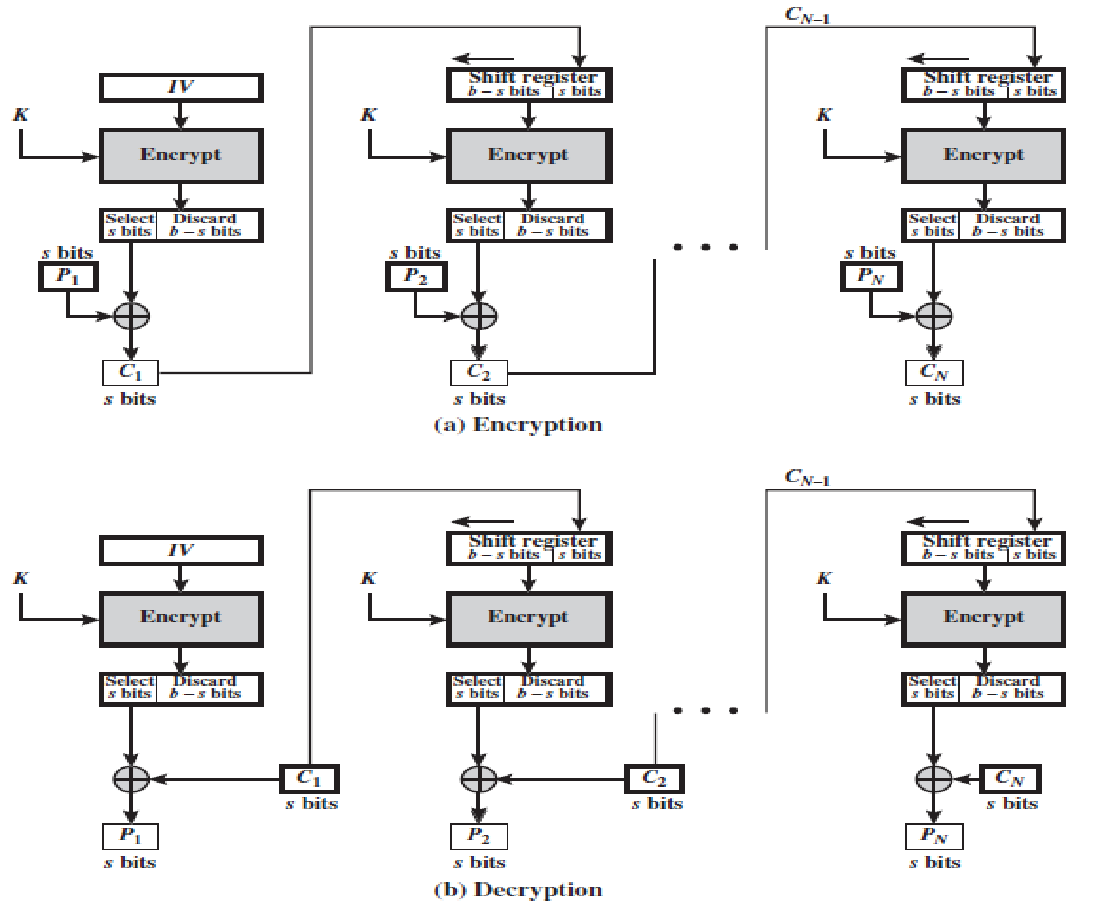Encryption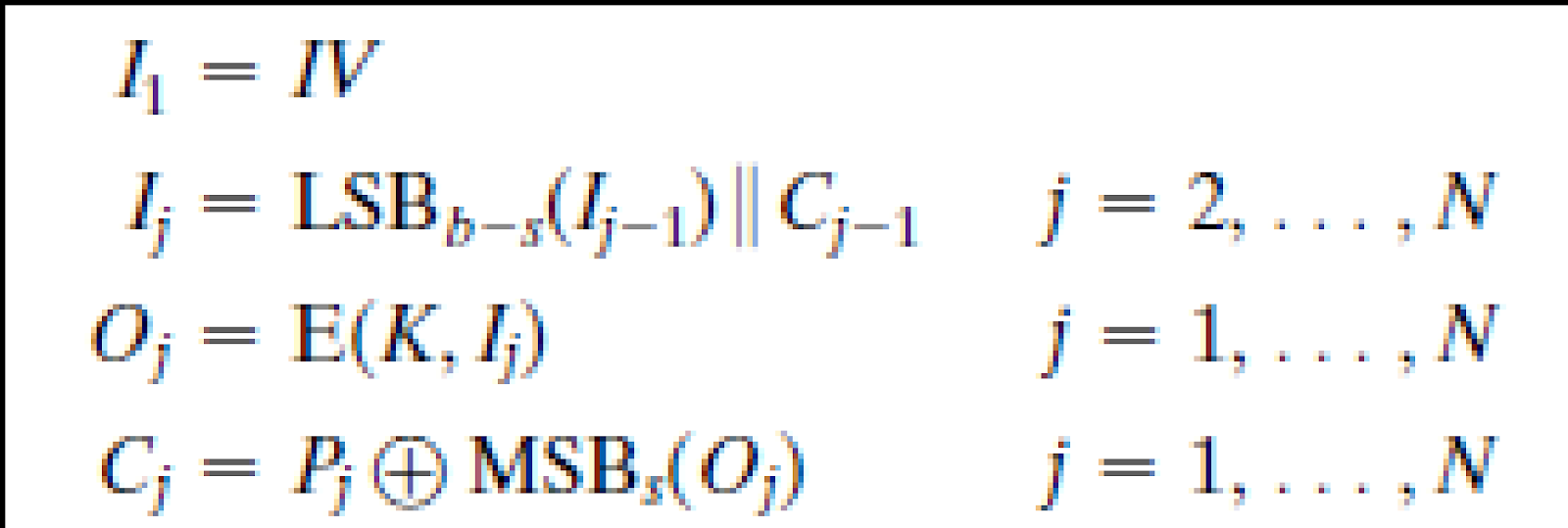Decryption